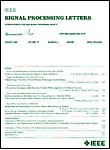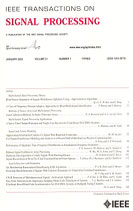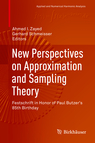Paulo J. S. G. Ferreira

## Superoscillations

It is commonly believed that a signal of bandwidth w Hertz cannot oscillate at frequencies higher than 2w Hertz. Yet, given any fixed bandwidth, there exist finite energy signals that oscillate in an arbitrarily fast way over arbitrarily long time intervals.

Such oscillations have been called superoscillations. The signals that contain them are called superoscillating signals. The articles in this section study several issues raised by the existence of superoscillating signals.

Dae Gwan Lee and Paulo J. S. G. Ferreira. Superoscillations with Optimal Numerical Stability. IEEE Signal Processing Letters, vol. 21, no. 12, pp. 1443-1447, Dec. 2014.A bandlimited signal can oscillate at a rate faster than its bandlimit. This phenomenon, called "superoscillation", has applications e.g. in superresolution and superdirectivity. The synthesis of superoscillations is a numerically difficult problem. We introduce time translation sigma as a design parameter and give an explicit closed formula for the condition number of the matrix of the problem, as a function of sigma. This enables us to determine the best possible condition number, which is several orders of magnitude better than otherwise achievable.

Dae Gwan Lee and Paulo J. S. G. Ferreira. Superoscillations with optimum energy concentration. IEEE Transactions on Signal Processing, vol. 62, no. 18, pp. 4857-4867, Sep. 2014.Oscillations of a bandlimited signal at a rate faster than the bandlimit are called "superoscillations" and have applications e.g. in superresolution and superdirectivity. The synthesis of superoscillating signals is a numerically difficult problem. Minimum energy superoscillatory signals seem attractive for applications because (i) the minimum-energy solution is unique (ii) it has the smallest energy cost (iii) it may yield a signal of the smallest possible amplitude. On the negative side, superoscillating functions of minimum-energy depend heavily on cancellation and give rise to expressions that have very large coefficients. Furthermore, these coefficients have to be found by solving equations that are very ill-conditioned. Surprisingly, we show that by dropping the minimum energy requirement practicality can be gained rather than lost. We give a method of constructing superoscillating signals that leads to coefficients and condition numbers that are smaller by several orders of magnitude than the minimum-energy solution, yet yields energies close to the minimum. In contrast with the minimum-energy method, which builds superoscillations by linearly combining functions with an ill-conditioned Gram matrix, our method combines orthonormal functions, the Gram matrix of which is obviously the identity. Another feature of the method is that it yields the superoscillatory signal that maximises the energy concentration in a given set, which may or may not include the superoscillatory segment.

Dae Gwan Lee and Paulo J. S. G. Ferreira. Direct construction of superoscillations. IEEE Transactions on Signal Processing, vol. 62, no. 12, pp. 3125-3134, June 2014.Oscillations of a bandlimited signal at a rate faster than its maximum frequency are called "superoscillations" and have been found useful e.g. in connection with superresolution and superdirectivity. We consider signals of fixed bandwidth and with a finite or infinite number of samples at the Nyquist rate, which are regarded as the adjustable signal parameters. We show that this class of signals can be made to superoscillate by prescribing its values on an arbitrarily fine and possibly nonuniform grid. The superoscillations can be made to occur at a large distance from the nonzero samples of the signal. We give necessary and sufficient conditions for the problem to have a solution, in terms of the nature of the two sets involved in the problem. Since the number of constraints can in general be different from the number of signal parameters, the problem can be exactly determined, underdetermined or overdetermined. We describe the solutions in each of these situations. The connection with oversampling and variational formulations is also discussed.

Dae Gwan Lee and Paulo J. S. G. Ferreira. Superoscillations of prescribed amplitude and derivative. IEEE Transactions on Signal Processing, vol. 62, no. 13, pp. 3371-3378, July 2014.Superoscillations occur when a bandlimited signal oscillates at a rate higher than its maximum frequency. We show that it is possible to construct superoscillations by constraining not only the value of the signal but also that of its derivative. This allows a better control of the shape of the superoscillations. We find that for any given bandwidth, no matter how small, there exists a unique signal of minimum energy that satisfies any combination of amplitude and derivative constraints, on a sampling grid as fine as desired. We determine the energy of the signal, for any grid, regular or irregular. When the set of derivative constraints is empty the results reduce to minimum energy interpolation. In the absence of amplitude constraints, we obtain pure derivative-constrained extremals. The flexibility gained by having two different types of constraints makes it possible to design superoscillations based only on amplitudes, based only on derivatives, or based on both. In the last case, the amplitude and derivative sampling grids can be interleaved or aligned. We explore this flexibility to build superoscillations that cost less energy. Illustrating examples are given.

Paulo J. S. G. Ferreira. Superoscillations. In: New Perspectives on Approximation and Sampling Theory: Festschrift in honor of Paul Butzer’s 85th birthday, Gerhard Schmeisser and Ahmed Zayed (Eds.). Springer, pp. 247-268, 2014.A band-limited function may oscillate faster than its maximum Fourier component, and it may do so over arbitrarily long intervals. The goal of this chapter is to discuss this phenomenon, which has been called "superoscillation". Although the theoretical interest in superoscillating functions is relatively recent, a number of applications are already known (in quantum physics, superresolution, subwavelength imaging and antenna theory). This chapter gives a brief account of how superoscillations appeared and developed and discusses their cost and some of their implications.

Paulo J. S. G. Ferreira and Armando J. Pinho. The natural scale of signals: pulse duration and superoscillations. In: Proceedings of the IEEE International Conference on Acoustics, Speech, and Signal Processing, ICASSP 2014, Florence, Italy, pp. 4176-4179, May 2014.Superoscillations are oscillations at frequencies above the maximum frequency in the signal spectrum. Signals of very small bandwidth can indeed oscillate at arbitrarily high frequencies, over arbitrarily long intervals. This work addresses the matter from a different angle, emphasizing scale and discussing the following question: can an arbitrarily narrow pulse be constructed by linearly combining arbitrarily wider pulses? The connection with superoscillations and approximation theory is also discussed.

P. J. S. G. Ferreira, A. Kempf and M. J. C. S. Reis. Construction of Aharonov-Berry's superoscillations. Journal of Physics A: Mathematical and General, vol. 40, pp. 5141-5147, 2007.A simple method is described for constructing functions that superoscillate at an arbitrarily chosen wavelength scale. Our method is based on the technique of oversampled signal reconstruction. This allows us to explicitly demonstrate that the observed fragility of superoscillating wave functions is indeed mathematically closely connected to what in the communication theory community is known as the instability of oversampled signal reconstruction, confirming a previous conjecture. This is of potential interest, for example, concerning the understanding of the practical difficulties in experimentally producing superoscillatory wave functions.

P. J. S. G. Ferreira and A. Kempf. Superoscillations: Faster than the Nyquist Rate. IEEE Transactions on Signal Processing, vol. 54, no. 10, pp. 3732-3740, Oct. 2006.For any fixed bandwidth there exist finite energy signals that oscillate arbitrarily fast over arbitrarily long time intervals. These localized fast transients or superoscillations can only occur in signals which possess amplitudes of widely different scales. This paper investigates the required dynamical range and energy (squared L_2 norm) as a function of the superoscillation's frequency, number, and maximum derivative. It briefly discusses some of the implications of superoscillating signals, in reference to information theory and time-frequency analysis, for example. It shows, among other things, that the required energy grows exponentially with the number of superoscillations, and polynomially with the reciprocal of the bandwidth or the reciprocal of the superoscillations' period.

A. Kempf and P. J. S. G. Ferreira. Unusual properties of superoscillating particles. Journal of Physics A: Mathematical and General, vol. 37, pp. 12067-12076, 2004.It has been found that differentiable functions can locally oscillate on length scales that are much smaller than the smallest wave length contained in their Fourier spectrum. This phenomenon has been called superoscillation. Here, we consider the case of superoscillations in quantum mechanical wave functions. We find that superoscillations in wave functions lead to unusual phenomena that are of measurement theoretic, thermodynamic and information theoretic interest.

P. J. S. G. Ferreira and A. Kempf. The energy expense of superoscillations. In: Signal Processing XI — Theories and Applications. Proceedings of EUSIPCO-2002, XI European Signal Processing Conference, vol. II, Toulouse, France, pp. 347-350, Sep. 2002.

For any fixed bandwidth, there are finite energy signals which oscillate arbitrarily fast on arbitrarily long, finite time intervals. This paper investigates such signals, and their implications in reference to the transmission of information through low bandwidth channels.